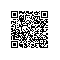# 独家 | 为你介绍7种流行的线性回归收缩与选择方法（附代码）

### 设置和数据加载

Hastie等人的网站

http://web.stanford.edu/~hastie/ElemStatLearn/

# Import necessary modules and set options

import pandas as pd

import numpy as np

import itertools

from sklearn.linear_model import LinearRegression, RidgeCV, LassoCV, ElasticNetCV, LarsCV

from sklearn.cross_decomposition import PLSRegression

from sklearn.decomposition import PCA

from sklearn.pipeline import Pipeline

from sklearn.model_selection import GridSearchCV

import warnings

warnings.filterwarnings("ignore")

data = pd.read_csv("prostate_data", sep = "\t")

# Train-test split

y_train = np.array(data[data.train == "T"]['lpsa'])

y_test = np.array(data[data.train == "F"]['lpsa'])

X_train = np.array(data[data.train == "T"].drop(['lpsa', 'train'], axis=1))

X_test = np.array(data[data.train == "F"].drop(['lpsa', 'train'], axis=1))

### 线性回归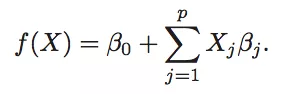p个特征系数β，每个变量一个，表示它们对目标的影响;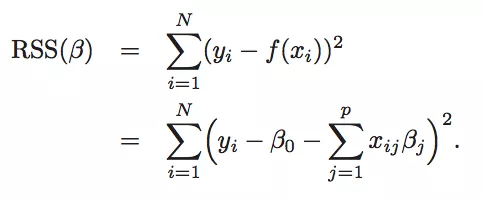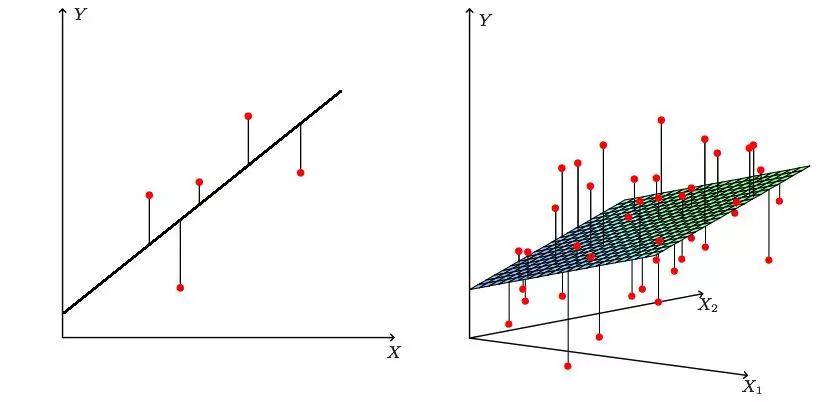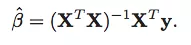### 偏差-方差权衡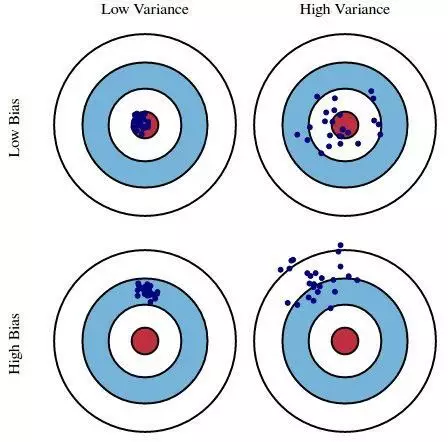linreg_model = LinearRegression(normalize=True).fit(X_train, y_train)

linreg_prediction = linreg_model.predict(X_test)

linreg_mae = np.mean(np.abs(y_test - linreg_prediction))

linreg_coefs = dict(

zip(['Intercept'] + data.columns.tolist()[:-1],

np.round(np.concatenate((linreg_model.intercept_, linreg_model.coef_),

axis=None), 3))

)

print('Linear Regression MAE: {}'.format(np.round(linreg_mae, 3)))

print('Linear Regression coefficients:')

linreg_coefs

### 最佳子集回归

results = pd.DataFrame(columns=['num_features', 'features', 'MAE'])

# Loop over all possible numbers of features to be included

for k in range(1, X_train.shape + 1):

# Loop over all possible subsets of size k

for subset in itertools.combinations(range(X_train.shape), k):

subset = list(subset)

linreg_model = LinearRegression(normalize=True).fit(X_train[:, subset], y_train)

linreg_prediction = linreg_model.predict(X_test[:, subset])

linreg_mae = np.mean(np.abs(y_test - linreg_prediction))

results = results.append(pd.DataFrame([{'num_features': k,

'features': subset,

'MAE': linreg_mae}]))

# Inspect best combinations

results = results.sort_values('MAE').reset_index()

# Fit best model

best_subset_model = LinearRegression(normalize=True).fit(X_train[:, results['features']], y_train)

best_subset_coefs = dict(

zip(['Intercept'] + data.columns.tolist()[:-1],

np.round(np.concatenate((best_subset_model.intercept_, best_subset_model.coef_), axis=None), 3))

)

print('Best Subset Regression MAE: {}'.format(np.round(results['MAE'], 3)))

print('Best Subset Regression coefficients:')

best_subset_coefs


### 岭回归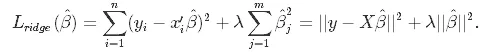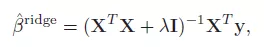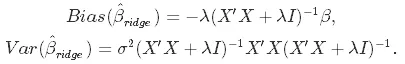ridge_cv = RidgeCV(normalize=True, alphas=np.logspace(-10, 1, 400))

ridge_model = ridge_cv.fit(X_train, y_train)

ridge_prediction = ridge_model.predict(X_test)

ridge_mae = np.mean(np.abs(y_test - ridge_prediction))

ridge_coefs = dict(

zip(['Intercept'] + data.columns.tolist()[:-1],

np.round(np.concatenate((ridge_model.intercept_, ridge_model.coef_),

axis=None), 3))

)

print('Ridge Regression MAE: {}'.format(np.round(ridge_mae, 3)))

print('Ridge Regression coefficients:')

ridge_coefs



### LASSO

Lasso，或最小绝对收缩和选择算子，在本质上与岭回归非常相似。它也为损失函数的非零系数增加了一个惩罚，但与惩罚平方系数之和（所谓的L2惩罚）的岭回归不同，LASSO惩罚它们的绝对值之和（L1惩罚）。因此，对于λ的高值，许多系数在LASSO下完全归零，在岭回归中从未如此。

LASSO的损失函数如下：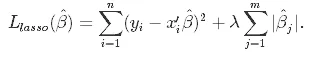lasso_cv = LassoCV(normalize=True, alphas=np.logspace(-10, 1, 400))

lasso_model = lasso_cv.fit(X_train, y_train)

lasso_prediction = lasso_model.predict(X_test)

lasso_mae = np.mean(np.abs(y_test - lasso_prediction))

lasso_coefs = dict(

zip(['Intercept'] + data.columns.tolist()[:-1],

np.round(np.concatenate((lasso_model.intercept_, lasso_model.coef_), axis=None), 3))

)

print('LASSO MAE: {}'.format(np.round(lasso_mae, 3)))

print('LASSO coefficients:')

lasso_coefs

### 弹性网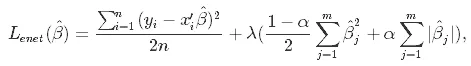elastic_net_cv = ElasticNetCV(normalize=True, alphas=np.logspace(-10, 1, 400),

l1_ratio=np.linspace(0, 1, 100))

elastic_net_model = elastic_net_cv.fit(X_train, y_train)

elastic_net_prediction = elastic_net_model.predict(X_test)

elastic_net_mae = np.mean(np.abs(y_test - elastic_net_prediction))

elastic_net_coefs = dict(

zip(['Intercept'] + data.columns.tolist()[:-1],

np.round(np.concatenate((elastic_net_model.intercept_,

elastic_net_model.coef_), axis=None), 3))

)

print('Elastic Net MAE: {}'.format(np.round(elastic_net_mae, 3)))

print('Elastic Net coefficients:')

elastic_net_coefs

### 最小角度回归

LAR_cv = LarsCV(normalize=True)

LAR_model = LAR_cv.fit(X_train, y_train)

LAR_prediction = LAR_model.predict(X_test)

LAR_mae = np.mean(np.abs(y_test - LAR_prediction))

LAR_coefs = dict(

zip(['Intercept'] + data.columns.tolist()[:-1],

np.round(np.concatenate((LAR_model.intercept_, LAR_model.coef_), axis=None), 3))

)

print('Least Angle Regression MAE: {}'.format(np.round(LAR_mae, 3)))

print('Least Angle Regression coefficients:')

LAR_coefs


### 主成分回归


regression_model = LinearRegression(normalize=True)

pca_model = PCA()

pipe = Pipeline(steps=[('pca', pca_model), ('least_squares', regression_model)])

param_grid = {'pca__n_components': range(1, 9)}

search = GridSearchCV(pipe, param_grid)

pcareg_model = search.fit(X_train, y_train)

pcareg_prediction = pcareg_model.predict(X_test)

pcareg_mae = np.mean(np.abs(y_test - pcareg_prediction))

n_comp = list(pcareg_model.best_params_.values())

pcareg_coefs = dict(

zip(['Intercept'] + ['PCA_comp_' + str(x) for x in range(1, n_comp + 1)],

np.round(np.concatenate((pcareg_model.best_estimator_.steps.intercept_,

pcareg_model.best_estimator_.steps.coef_), axis=None), 3))

)

print('Principal Components Regression MAE: {}'.format(np.round(pcareg_mae, 3)))

print('Principal Components Regression coefficients:')

pcareg_coefs

### 偏最小二乘法

pls_model_setup = PLSRegression(scale=True)

param_grid = {'n_components': range(1, 9)}

search = GridSearchCV(pls_model_setup, param_grid)

pls_model = search.fit(X_train, y_train)

pls_prediction = pls_model.predict(X_test)

pls_mae = np.mean(np.abs(y_test - pls_prediction))

pls_coefs = dict(

zip(data.columns.tolist()[:-1],

np.round(np.concatenate((pls_model.best_estimator_.coef_), axis=None), 3))

)

print('Partial Least Squares Regression MAE: {}'.format(np.round(pls_mae, 3)))

print('Partial Least Squares Regression coefficients:')

pls_coefs

### 回顾与结论

• 最佳子集回归迭代所有可能的特征组合以选择最佳特征组合;
• 岭回归惩罚平方系数值（L2惩罚），强制它们很小;
• LASSO惩罚系数的绝对值（L1惩罚），这可以迫使它们中的一些精确为零;
• 弹性网结合了L1和L2的惩罚，享受了Ridge和Lasso的精华;
• 最小角度回归适用于子集和收缩之间：它迭代地工作，在每个步骤中添加其中一个特征的“某个部分”;
• 主成分回归执行PCA将原始特征压缩为一小部分新特征，然后将其用作预测变量;
• 偏最小二乘也将原始特征概括为较小的新特征子集，但与PCR不同，它也利用目标构建它们。

###### 来源
Hastie, T., Tibshirani, R., & Friedman, J. H. (2009). The elements of statistical learning: data mining, inference, and prediction. 2nd ed. New York: Springer.

https://www.datacamp.com/community/tutorials/tutorial-ridge-lasso-elastic-net

A Comparison of Shrinkage and Selection Methods for Linear Regression

https://towardsdatascience.com/a-comparison-of-shrinkage-and-selection-methods-for-linear-regression-ee4dd3a71f16

### 译者简介